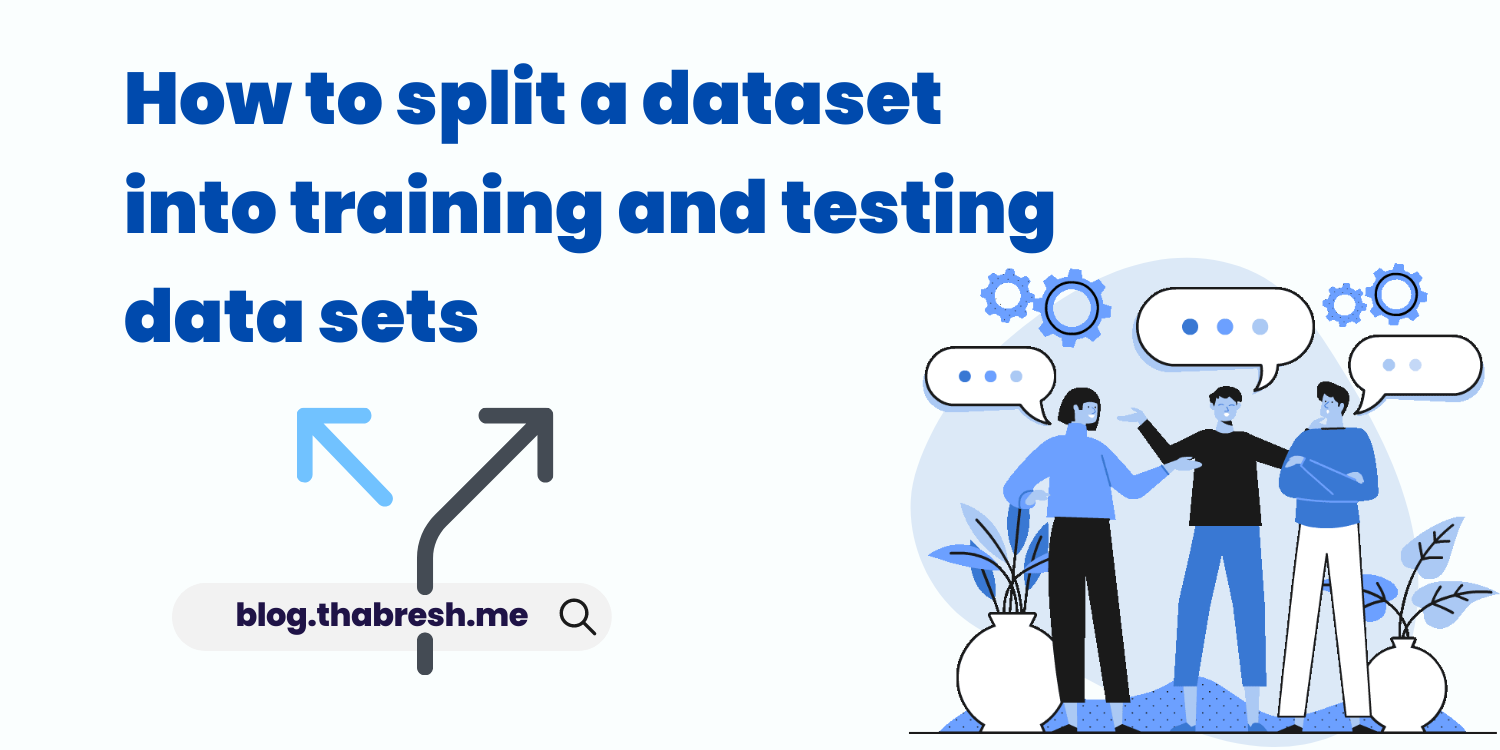# How to split a dataset into training and testing data sets for Machine Learning

Dataset split, training and testing dataset, scilit-learn library, machine learning, thabresh syed,data science# Introduction

Training and testing datasets are subsets of a larger dataset used in machine learning to develop and evaluate a model. The larger dataset is typically split into two subsets: the training set and the testing set.

## Training Dataset

The training dataset is used to fit the model, which involves finding the optimal parameters that best fit the data. The model is exposed to the training dataset and learns the relationship between the features and the target variable. The training set is used to train the model to generalize well on unseen data.

## Testing Dataset

The testing dataset is used to evaluate the performance of the trained model. The model is applied to the testing dataset to make predictions, and the predicted values are compared with the actual values in the testing dataset. The goal of the testing dataset is to measure how well the model generalizes to new, unseen data.

## Why we splitting datasets?

By splitting the dataset into training and testing subsets, we can estimate how well the model performs on new data. This is important because the ultimate goal of a machine learning model is to make accurate predictions on new data that was not seen during training.

# Splitting training and testing data

## train_test_split function

To split a dataset into training and testing datasets for machine learning, you can use the scikit-learn library's train_test_split function. Here's an example code snippet that shows how to split a dataset into training and testing sets:
```
from sklearn.model_selection import train_test_split

# Splitting the dataset into training and testing sets
X_train, X_test, y_train, y_test = train_test_split(X, y, test_size=0.2, random_state=42)

# X and y are the feature and target variables, respectively
# test_size is the proportion of the dataset that should be allocated for testing
# random_state is a parameter that ensures the splitting is done in a reproducible way
```
1. X and y represent the dataset's features and target variable, respectively.
2. train_test_split is a function from scikit-learn's model_selection module that splits the dataset into training and testing sets.
3. The test_size parameter specifies the proportion of the dataset that should be allocated for testing. In this case, 20% of the data is reserved for testing.
4. The random_state parameter specifies the seed used by the random number generator. This ensures that the data is split in a reproducible way.
5. X_train and y_train represent the training set's features and target variable, respectively.
6. X_test and y_test represent the testing set's features and target variable, respectively.

## Example

Let's say we have a dataset of 1000 housing prices, with features such as square footage, number of bedrooms, and location. We want to build a machine learning model that can predict the price of a house based on its features.

We can split the dataset into a training set and a testing set. For example, we can use 80% of the data (800 samples) for training the model and the remaining 20% (200 samples) for testing the model. We randomly assign the samples to the training and testing sets, so that both sets are representative of the overall dataset.

We can use the training set to fit a regression model that predicts the housing prices based on the features. We can then use the testing set to evaluate the performance of the model by making predictions on the testing set and comparing them to the actual prices.

If the model performs well on the testing set, we can have confidence that it will generalize well to new, unseen data. If the model performs poorly on the testing set, we may need to re-evaluate our model or collect more data to improve the model's performance.
```
from sklearn.model_selection import train_test_split
import pandas as pd

# Load the dataset into a pandas dataframe

# Split the dataset into features and target variable
X = df.drop('price', axis=1)
y = df['price']

# Split the dataset into training and testing sets
X_train, X_test, y_train, y_test = train_test_split(X, y, test_size=0.2, random_state=1)

# Print the shape of the training and testing sets
print('Training set:', X_train.shape, y_train.shape)
print('Testing set:', X_test.shape, y_test.shape)
```

In this example, we first load the housing dataset into a pandas dataframe. We then split the dataset into features (X) and target variable (y). We use the train_test_split function from the sklearn.model_selection module to split the dataset into training and testing sets. The test_size parameter specifies the percentage of the data to be used for testing, which is set to 20% in this example. The random_state parameter sets the seed for the random number generator to ensure reproducibility of the results.

Finally, we print the shape of the training and testing sets to confirm that the data has been split correctly. We can use the X_train, X_test, y_train, and y_test variables in our machine learning model to fit the model on the training data and evaluate its performance on the testing data.

# Conclusion

Splitting a dataset into training and testing sets is a crucial step in machine learning. The training set is used to train a model, while the testing set is used to evaluate the model's performance. This helps to ensure that the model generalizes well to new, unseen data.

The scikit-learn library provides a convenient function called train_test_split that can be used to split a dataset into training and testing sets in Python. By splitting the dataset into training and testing sets, we can evaluate the performance of our model and make any necessary adjustments before deploying the model in the real world.

Related Posts

Thank You So Much for Reading How to split a dataset into training and testing data sets for Machine Learning Article.